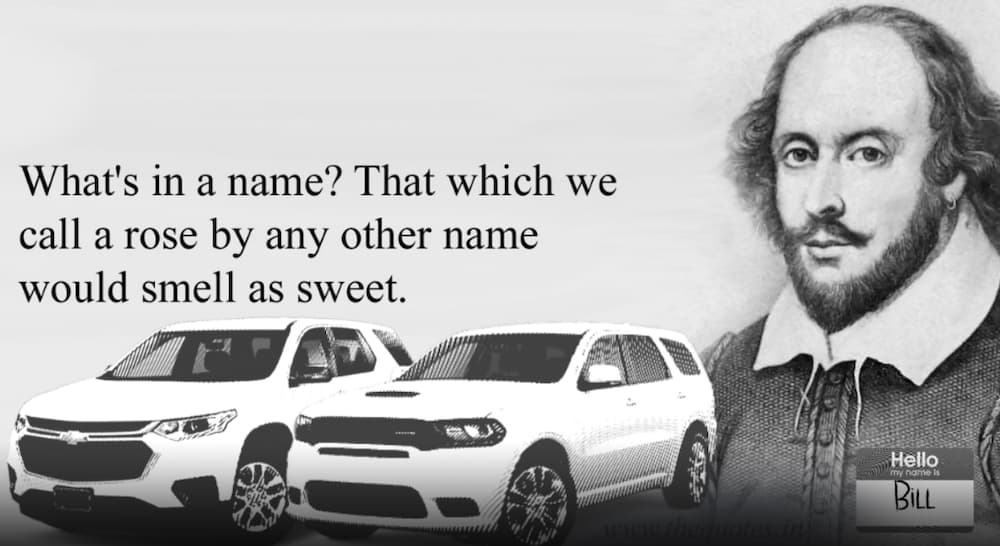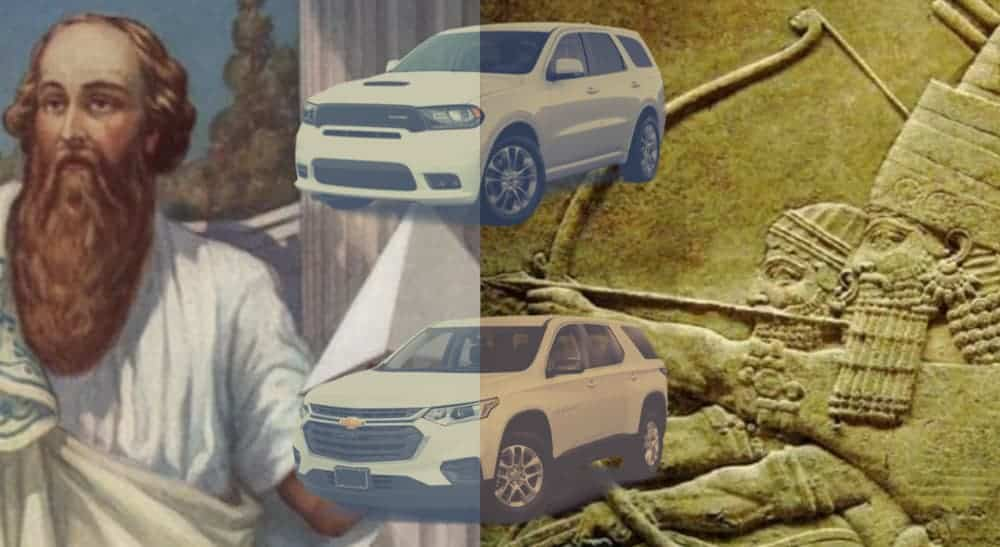# The Traverse vs Durango: By the Numbers

0
421Choosing the right midsize SUV is hard – there’s a reason most people request a few days off from work in order to pick the best option. Sure, you could take the time to look at the different spec sheets for various models, compare what each one has to offer in terms of their size, cargo capacity, engine power, fuel economy, etc. You could then figure out just what you need from a vehicle like that to make your life easier, and cross options off as you realize they’re not right for you. This would be a great way to choose between two different SUVs, like the 2020 Chevy Traverse vs 2020 Dodge Durango, for example.

Or, you could skip all that boring stuff and do what makes sense: use numerology and the names of different vehicles to figure out which one is right for you. I mean, we’re talking about SUVs here, so the simplest and most definitive way to choose the right model is by looking at their names and how they compare in different numerological systems. Makes sense to me…## What’s in a Name?

Before we get started, let’s take a moment to talk about why what we’re doing works. Any acolyte will tell you that there’s power in naming. It’s basically the first thing you learn, and it’s essentially Chapter One of any grimoire you find that’s actually worth a hot damn. But why is that?

By naming a thing, you give it focus; you provide form and function to it in a way that surpasses even its physical being. A door functions as a door because we call it that – same with a window. You can use either to enter or leave a room, but they are separate schemas in conceptual reality due to their names. So in giving something a name, you imbue it with its very essence. Therefore, we can look at the names of these two vehicles and pull from them the meaning and purpose they are meant to serve.## Pythagorean vs. Chaldean Numerology

There are numerous numerology systems, based on different alphabets and assigning numerical values to letters or words to derive an overall value for something. Since they’re tied to particular alphabets and languages, some won’t work for us since we’re looking at two vehicles with (more or less) English names. So that leaves us with two good options (I’m sure I could come up with more, but I’m not going to, so deal with it).

The Pythagorean numerology table is based on the teachings of the Greek mathematician and philosopher, Pythagoras, who lived about 2,500 years ago. He believed that numbers were the key to understanding all things, based largely on his interest in the harmonic vibrations of string instruments and the ability to understand them through numerical notations. The system based on his name is relatively simple: each letter has a corresponding value from 1-9 based on where it falls when listed.

So:

1 = a, j, s
2 = b, k, t
3 = c, l, u
4 = d, m, v
5 = e, n, w
6 = f, o, x
7 = g, p, y
8 = h, q, z
9 = i, r

Simple, right? Don’t worry too much about the fact that 4 gives us “DMV” or how 6 is represented by the “FOX.” Those are simply coincidences that rely on your mind looking for patterns in things. They’re not at all the key to a secret cabal ruling the world that you should spend the rest of your life searching for.

The other numerology system that works well with the English language is called the Chaldean system, named after the people that ruled Babylonia about 2,500 years ago. This is sometimes also called the Babylonian system, and it’s based on frequencies of sounds made when speaking someone or something’s name aloud. It uses a system based on 1-8, without 9 because in the Chaldean system, 9 is connected to the infinite. The order is a little more complicated with this system because of differences in language over the last couple thousand years.

Its values work out as:

1 = a, i, j, q, y
2 = b, k, r
3 = c, g, l, s
4 = d, m, t
5 = e, h, n, x
6 = u, v, w
7 = o, z
8 = f, p

Again, don’t worry too much about some patterns there, like the appearance of Oz or the fact that DMZ for 4 has now been replaced by DMT, the abbreviation for Dimethyltryptamine, a powerful hallucinogenic drug. These things aren’t related, and any connections you find between them are simply your mind adapting to a new understanding of powerful symbols and the ways that language actualizes the source code your brain subconsciously processes all day long.

## Pythagorean: Chevy Traverse

Now that we’ve gotten all that out of the way let’s get to the good stuff. Using the Pythagorean system, the Chevy Traverse comes up as the following:

C(3) + H(8) + E(5) + V(4) + R(9) + O(6) + L(3) + E(5) + T(2) = 45
T(2) + R(9) + A(1) + V(4) + E(5) + R(9) + S(1) + E(5) = 36

We then add those numbers together until we get a single digit:

45 -> 4+5 = 9
36 -> 3+6 = 9
9+9 = 18
1+8 = 9

That’s a lot of 9s! This is a very universe-oriented number, expressive of tremendous empathy and compassion, as well as understanding. So the Traverse is a great choice for anyone looking to make a change and help other people.

## Pythagorean: Dodge Durango

Now let’s do the same for the Durango:

D(4) + O(6) + D(4) + G(7) + E(5) = 26
D(4) + U(3) + R(9) + A(1) + N(5) + G(7) + O(6) = 35

And we again simplify it to a single digit:

26 -> 2+6 = 8
35 -> 3+5 = 8
8+8 = 16
1+6 = 7

So our number for the Durango (despite all those 8s) is 7. This is the number of the intellect, of the scientific mind and the pursuit of knowledge. That means the Dodge Durango is a terrific choice for anyone interested in the search for meaning in this universe.

## Chaldean: Chevy Traverse

Just to be sure we really know what’s what, let’s do this again for the Chaldean system for both vehicles. Here we go!

C(3) + H(5) + E(5) + V(6) + R(2) + O(7) + L(3) + E(5) + T(4) = 40
T(4) + R(2) + A(1) + V(6) + E(5) + R(2) + S(3) + E(5) = 28
40 -> 4+0 = 4
28 -> 2+8 = 10; 1+0 = 1
4+1 = 5

So, based on the Chaldean system, we see the Traverse has the number 5. This is an expression of travel and experience, with a strong sense of adventure. That means the Chevy Traverse is an excellent choice for anyone looking to leave the road behind.

## Chaldean: Dodge Durango

Finally, we’ll run the numbers on the Durango now using the Chaldean system.

D(4) + O(7) + D(4) + G(3) + E(5) = 23
D(4) + U(6) + R(2) + A(1) + N(5) + G(3) + O(7) = 28
23 -> 2+3 = 5
28 -> 2+8 = 10; 1+0 = 1
5+1 = 6

So, here we see the Chaldean number for the Durango is 6. This number expresses a strong sense of morality and right/wrong, with a desire to help others and sort out problems. That means the Durango is a great option for Superman or someone interested in a service profession where you get to help others a lot.

## The Results

Two systems, two vehicles, four different numbers. The point? Numerology is BS, and the only numbers that matter are the vehicle specs. So go look at them and decide for yourself what vehicle is right for you. Anyone that says they can use numbers, tea leaves, or your “sun sign” to help you make decisions in life is just trying to rip you off.

Editor’s Note: We received a very helpful numerology reading from this writer that assisted us through some tough times. Therefore, we recommend you ignore that last paragraph and make all your decisions based on the perceived positions of astronomical bodies that are nowhere near where they appear from our vantage point. Also, numbers; numbers are magic! Thank you.

SHARE